# Free Coordinate Grid Worksheets

📆 20 May 2022
🔖 Other Category
📂 Gallery Type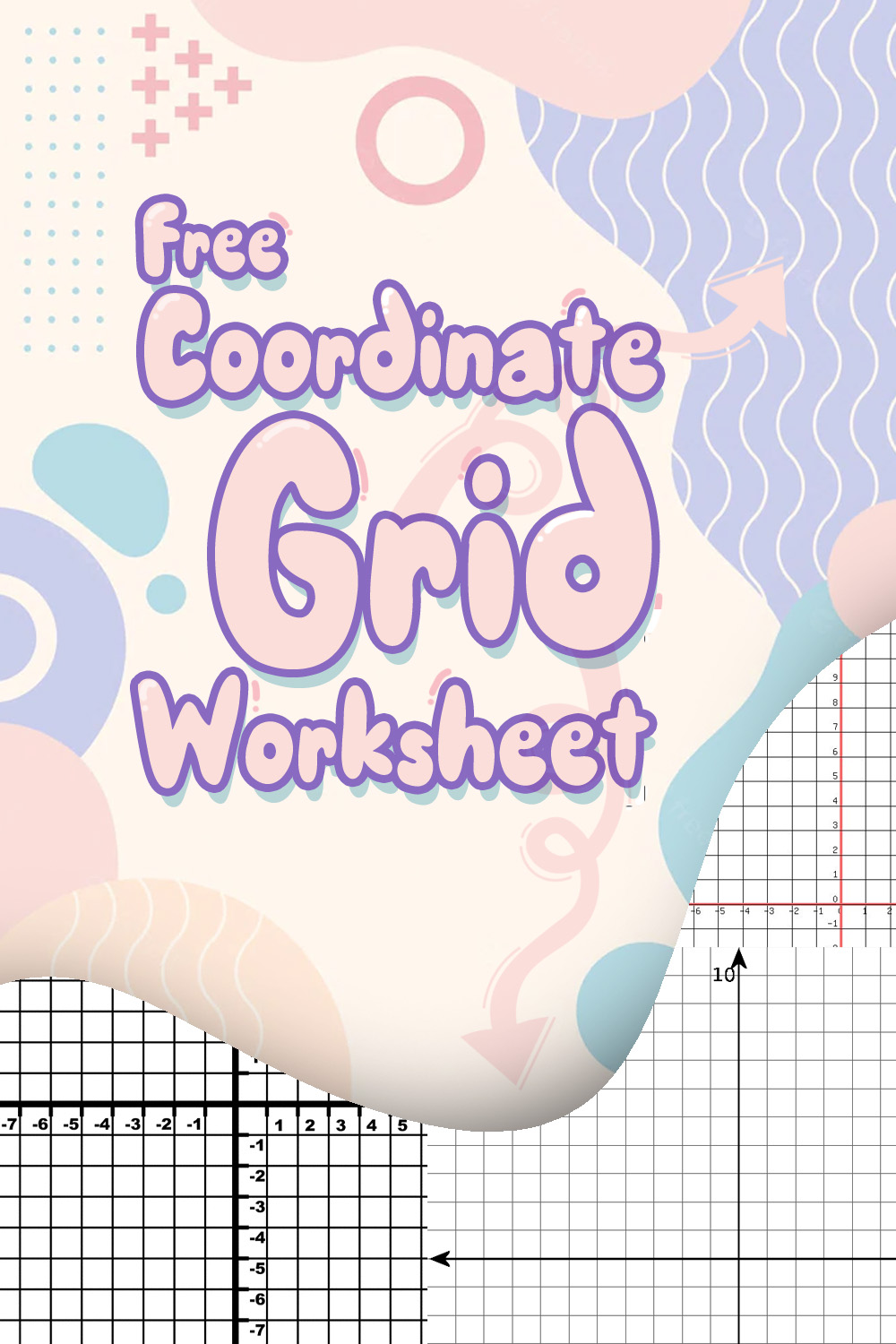9 Images of Coordinate Grid Worksheets

Free Coordinate Grid Worksheets is great to create activity for your student to learn and differentiate between positive and negative number. Download it now!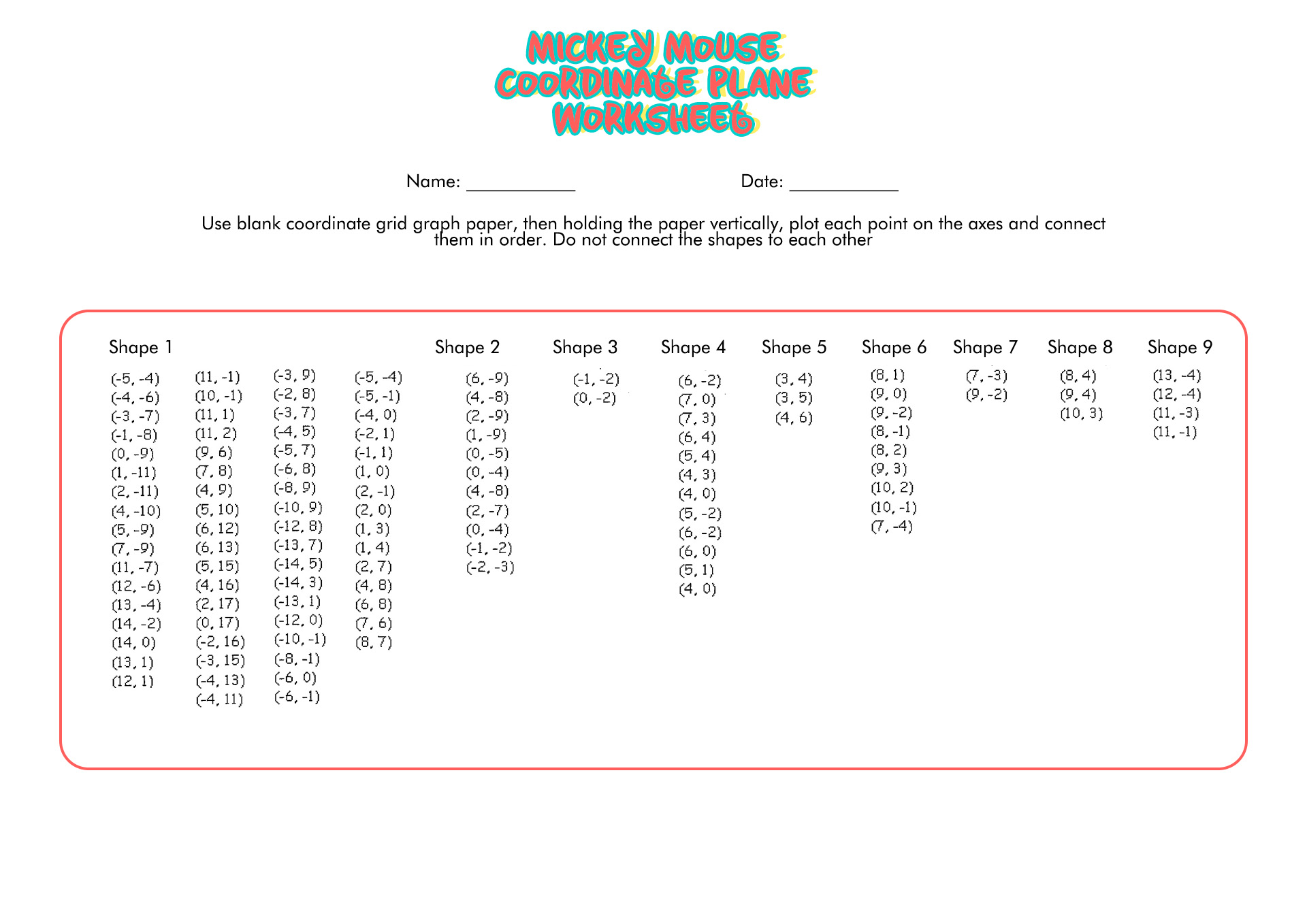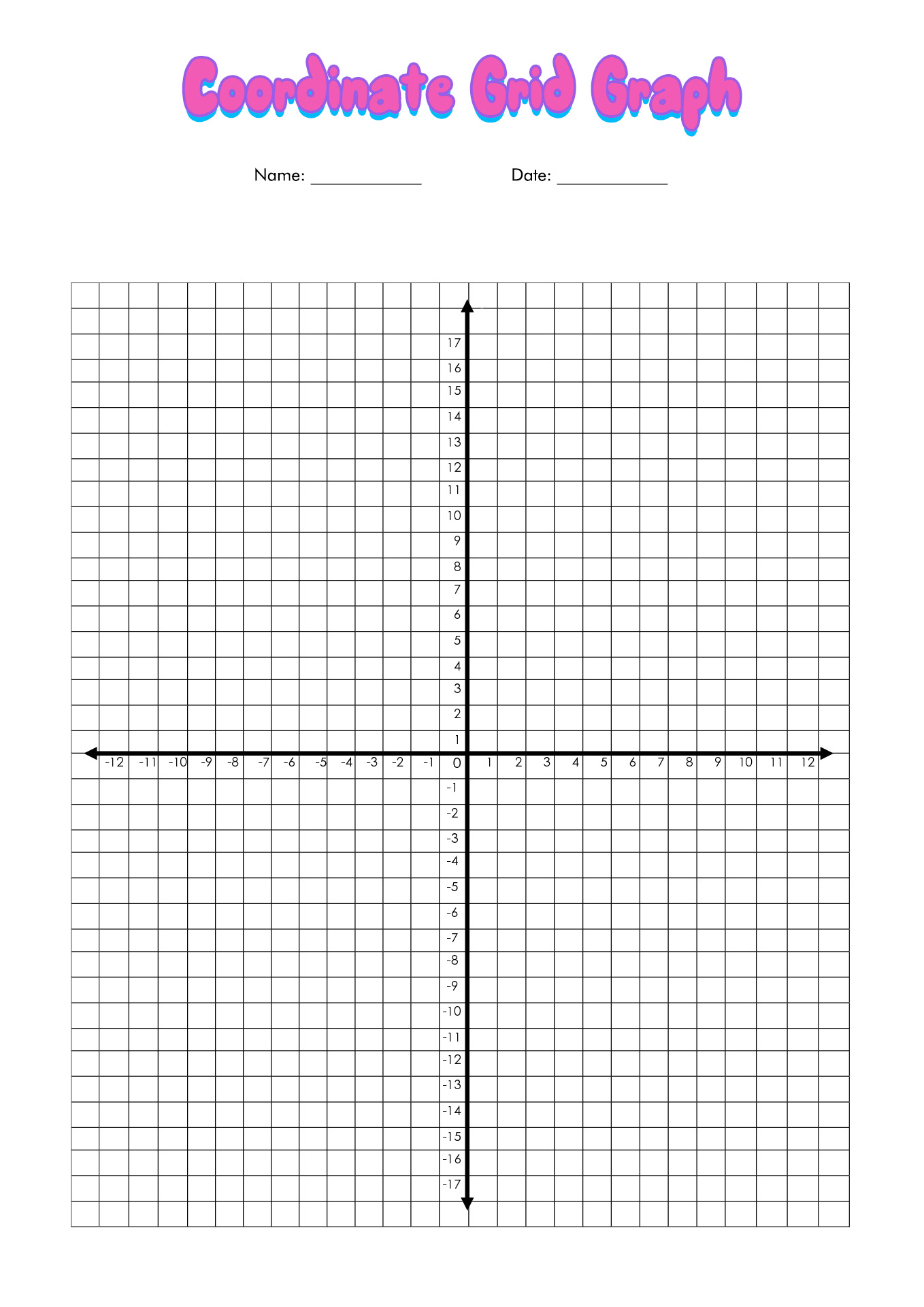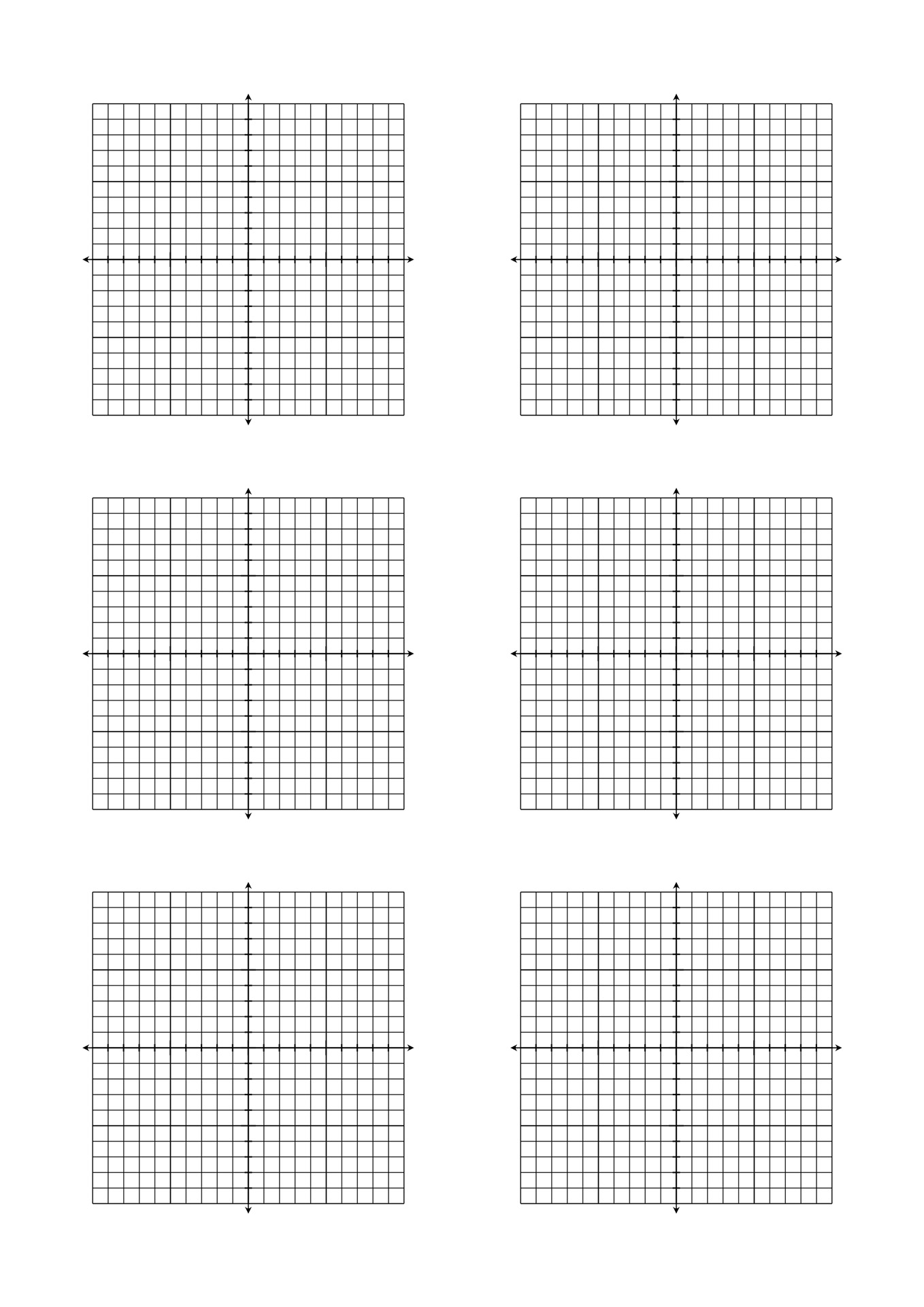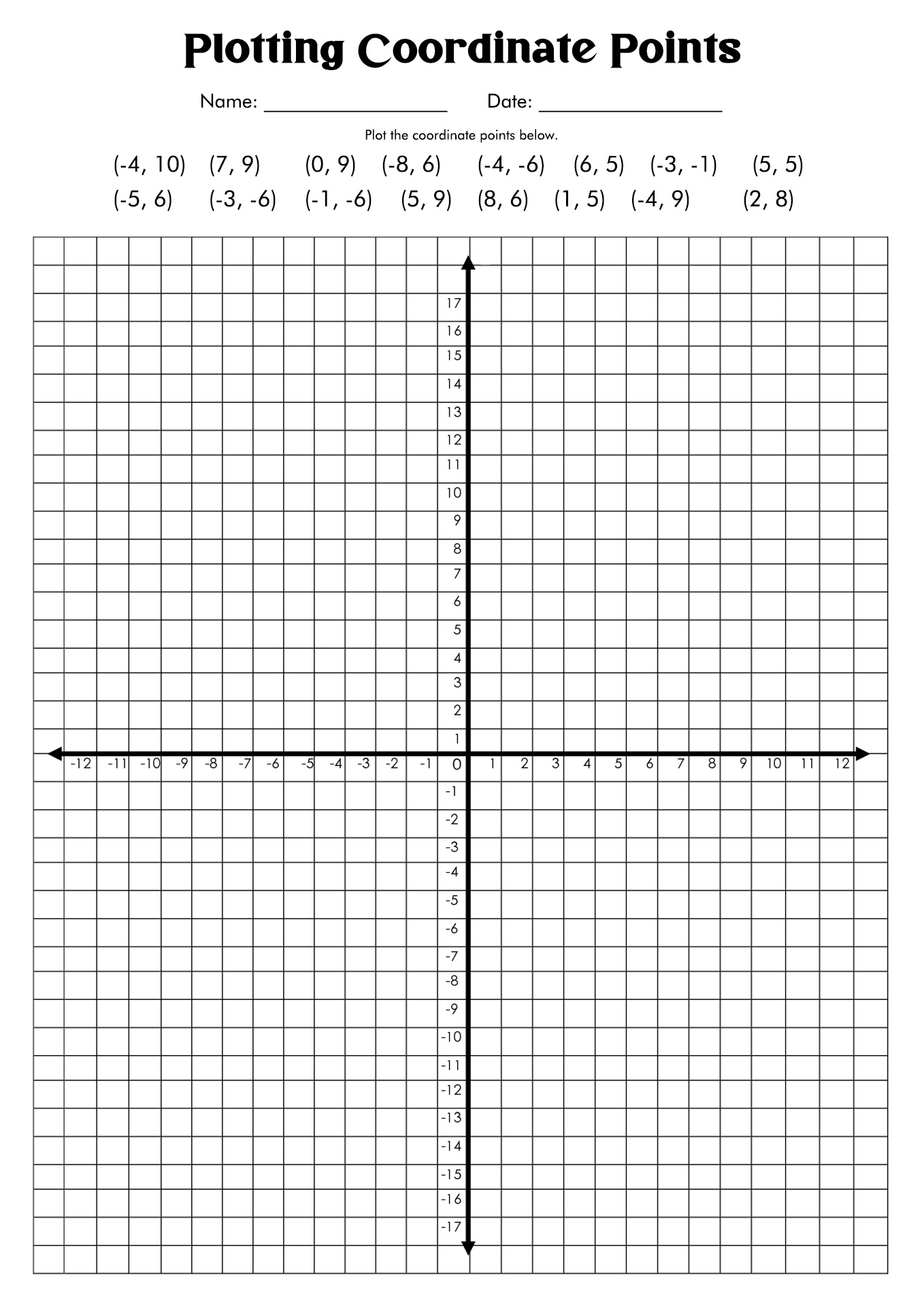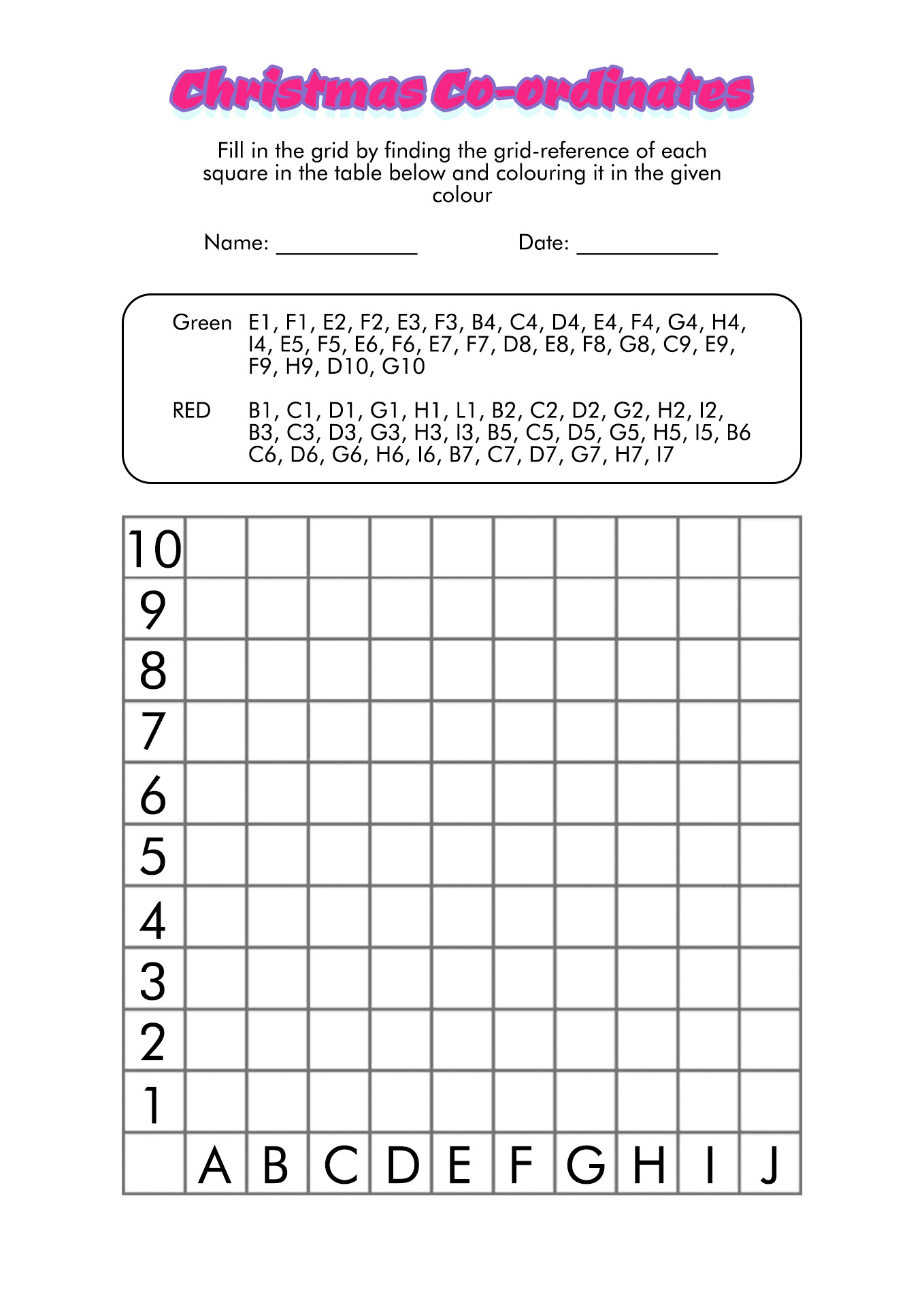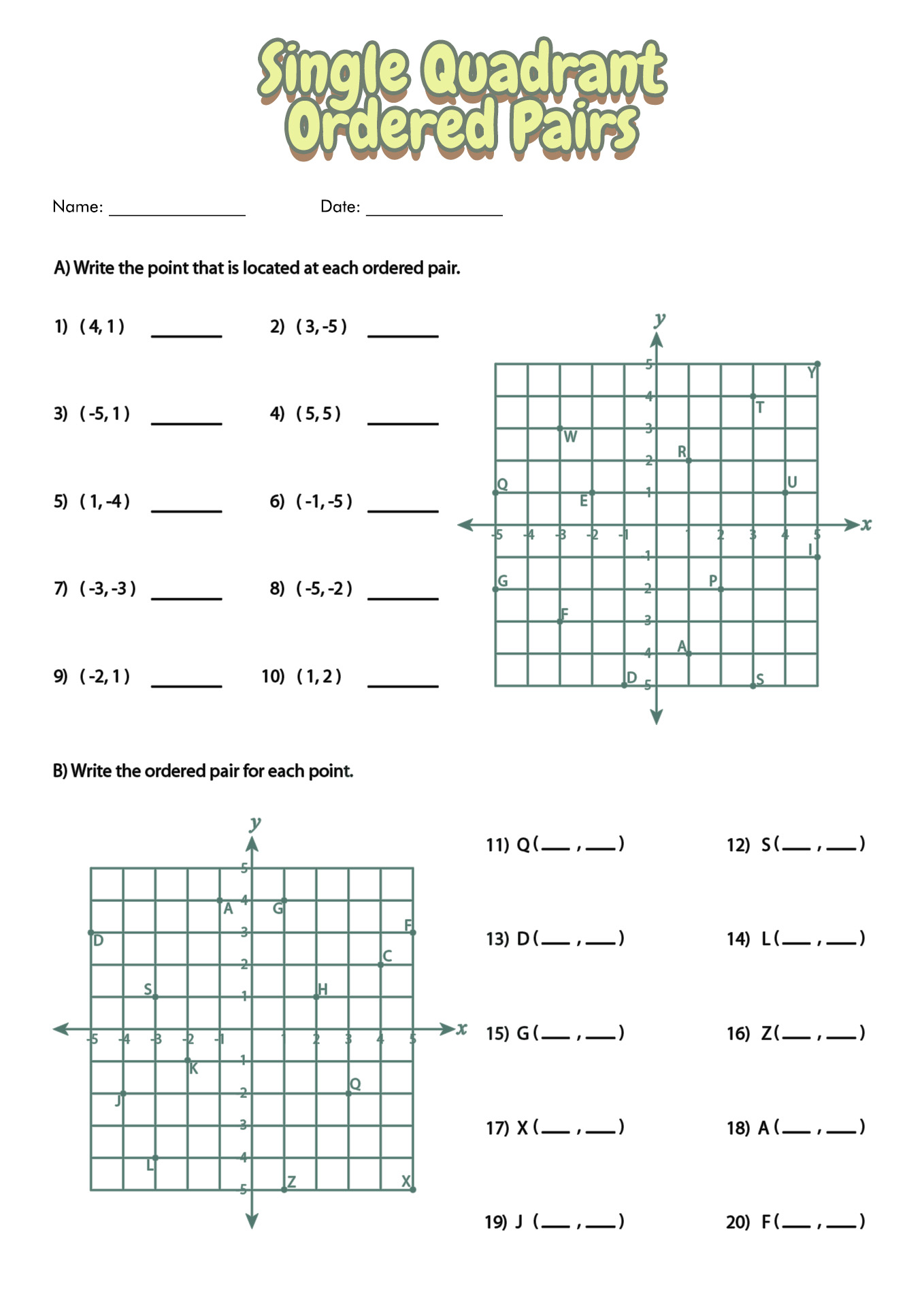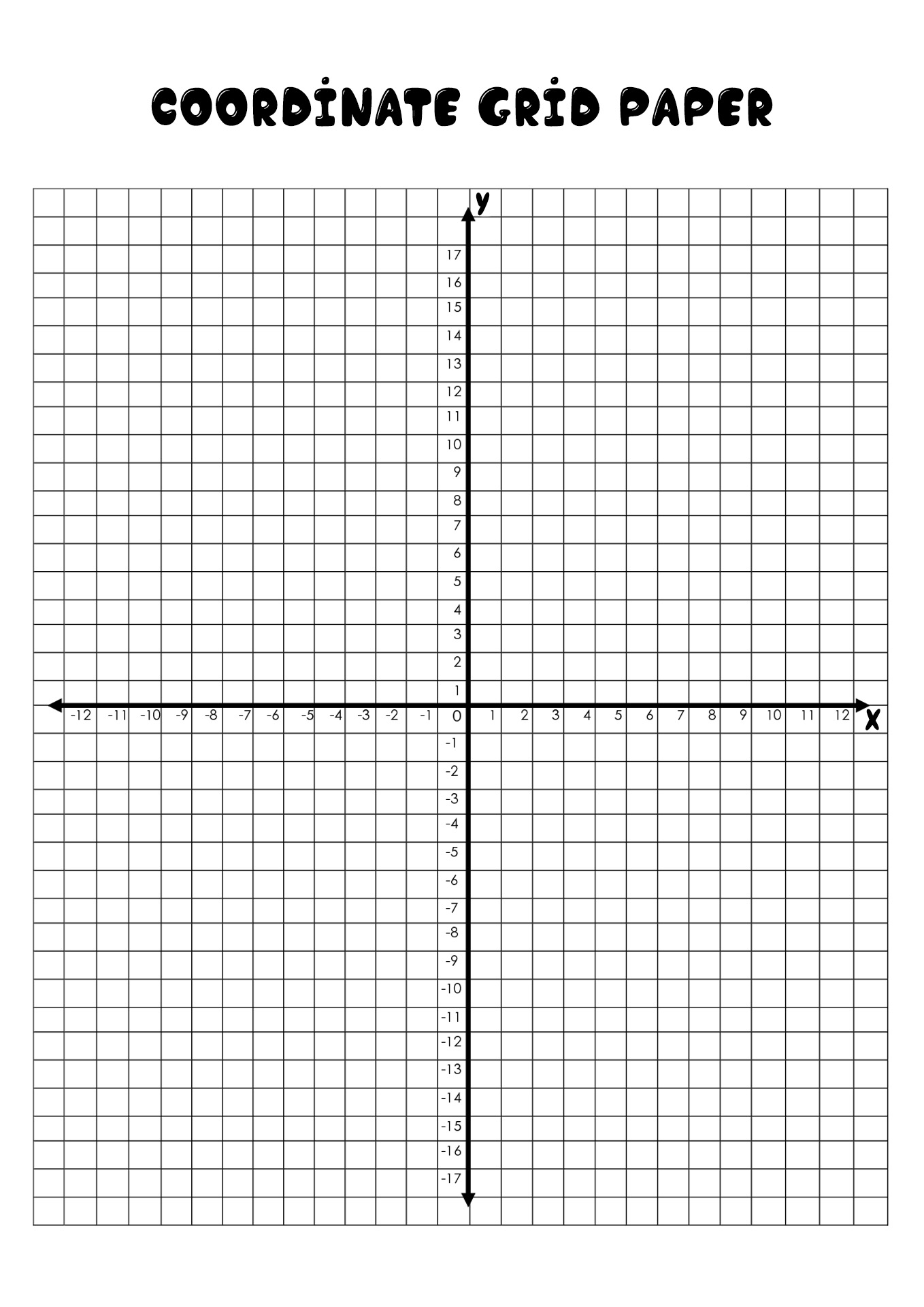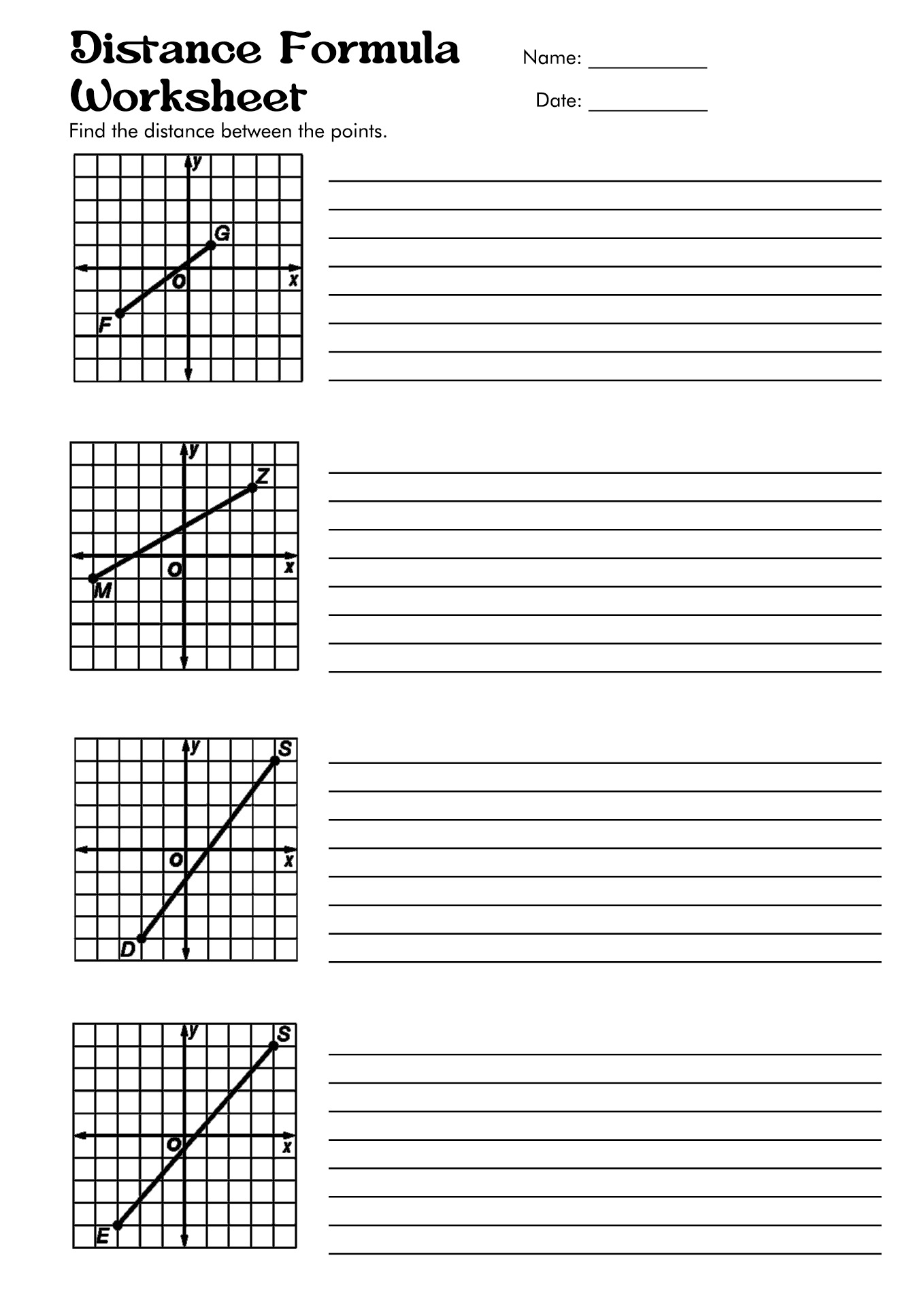Determine your successful math lesson with these Free Coordinate grid Worksheets!

Summary: The coordinate grid also known as the coordinate plane is the coordinate system is a two-dimensional surface that consists of two lines (axes). The horizontal axis in the coordinate grid is shown by the letter 'x' (x-axis) and the vertical axis is shown by the letter 'y' (y-axis). Learning a coordinate system is necessary for students because it will prepare them to learn more complicated mathematical subjects.

### What is A Coordinate Grid?

The coordinate system consists of points, lines or surfaces with unique coordination. The coordinate system is a crucial subject in mathematics. It is even the central subject that students need to learn at school. Mastering a coordinate system will help the students to understand algebra and geometry through the graphs of lines and curves. The coordinate grid, also known as the coordinate plane, is a two-dimensional surface made up of two lines and used to graph points, lines and other objects.

### What are The Types of Coordinate Systems?

There are three types of coordinate systems that are popular:

• The Cartesian Coordinate System: This coordinate system uses a coordinate plane with two crosswise axes (x and y) to locate the points. The x-axis stretches horizontally, and the y-axis runs vertically.
• The Polar Coordinate System: This coordinate system uses a coordinate plane with a single radial axis (r) and a single angular axis (Î). The points in the polar coordinate system are determined by the radius (r) and angle (Î).
• The Spherical Coordinate System: This coordinate system uses a three-dimensional coordinate system with a single radial axis (r), and two angular axes (Î) and Ïƒ, The points in the spherical coordinate system are determined by their radius (r), angles (Î), and Ïƒ.

### What are the Parts of The Coordinate Grid?

The teacher should introduce the parts of the coordinate grid to the students before diving into the lesson. This introduction will help the students to understand the topic they will learn. The teacher can use the Free Coordinate Grid Worksheets to introduce the children to the coordinate system. Here is a list of coordinate grid characteristics:

• The map on the coordinate system uses a horizontal and vertical grid to show information about the object's location.
• The coordinate grid has a horizontal axis, a vertical axis and a number of lines that intersects at right angles.
• The horizontal axis is represented by the letter 'x' (x-axis)
• The vertical axis is represented by the letter 'y' (y-axis).
• The point where the two axes cross is the origin.
• The crossing between the two axes divides the coordinate grid into four areas (quadrants).
• Ordered pairs are the location on the coordinate system.
• In ordered pair, the first value is the x-coordinate and the second value is the y-coordinate.

### How to Plot an Ordered Pair in Coordinate Plane?

There are three steps to plot an ordered pair (x,y) in the coordinate plane:

1. Pick the x-coordinate by starting from the origin and moving horizontally to the direction of the x-axis. If the x-coordinate is positive, move to the right, yet if the x-coordinate is negative, you should move to the left.
2. Pick the y-coordinate by starting from the x-coordinate and moving vertically to the direction of the y-axis. If the y-coordinate is positive, move up, yet if the y-coordinate is negative, you should move down.
3. Draw a point at the finishing location, and label the point with the ordered pair.

### What are The Students' Objectives in Learning Coordinate System?

Before palling the lesson plan for the teaching coordinate system, the teachers should determine the goals of the study. Here are the objectives of the coordinate system lesson:

• The students know that the coordinate plane permits two different dimensions of an object to be shown with one tiny point.
• The students understand that many mathematical subjects they can learn with coordinate systems.
• The students understand that the number plane is used to describe the location on the grid.
• The students recognize the coordinate systems consist of a horizontal axis (x-axis) and a vertical axis (y-axis).
• The students learn that the intersection of the horizontal axis and the vertical axis creates four areas in the coordinate (quadrants).
•  The students understand that the horizontal axis and the vertical axis meet at the correct angle (reasoning).
• The students are able to identify the points of intersection of the two axes.

### Why do We Need to Learn Coordinate System?

Learning coordinate systems might be challenging for some students. Sometimes, they also do not have the motivation to study it. To motivate your students, you can tell them about the advantages of learning coordinate systems or show them some trivia about it. The coordinate system can help students to understand more complicated mathematics subjects. Learning coordinate system will open their future opportunities in college or when they seek career opportunities. The teacher can download and use the Free Coordinate Grid Worksheets to help the students understand the coordinate system. The worksheets are full of cute illustrations that excite students to learn.

The information, names, images and video detail mentioned are the property of their respective owners & source.

### Popular Categories

Have something to tell us about the gallery?

Submit